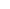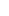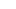###### Forex Trade Results July 22, 2022 – \$5,090
July 25, 2022July 26, 2022

# Free Margin Examples

## No Open Positions.

Let’s take a look at free margin examples.

You don’t have any open positions, what is your Free Margin?

### Step 1: Calculate Equity

If you don’t have any open position, calculating the Equity is easy.

`Equity = Account Balance + Floating Profits (or Losses) \$1,000 = \$1,000 + \$0`

The Equity would be the SAME as your Balance.

Since you don’t have any open positions, you don’t have any floating profits or losses.

### Step 2: Calculate Free Margin

If you don’t have any open positions, then the Free Margin is the SAME as the Equity.

`Free Margin = Equity - Used Margin \$1,000 = \$1,000 - \$0`

Since you don’t have any open positions, there is no margin being “used”.

This means that your Free Margin will be the same as your Balance and Equity.

## Example 2: Open a Long USD/JPY Position

Now let’s make it a bit more complicated by entering a trade!

Let’s say you have an account balance of \$1,000.

### Step 1: Calculate Required Margin

You want to go long USD/JPY and want to open 1 mini lot (10,000 units) position. The Margin Requirement is 4%.

How much margin (Required Margin) will you need to open the position?

Since USD is the base currency. this mini lot is 10,000 dollars, which means the position’s Notional Value is \$10,000.

`Required Margin = Notional Value x Margin Requirement \$400 = \$10,000 x .04`

Assuming your trading account is denominated in USD, since the Margin Requirement is 4%, the Required Margin will be \$400.

### Step 2: Calculate Used Margin

Aside from the trade we just entered, there aren’t any other trades open.

Since we just have a SINGLE position open, the Used Margin will be the same as Required Margin.

### Step 3: Calculate Equity

Let’s assume that the price has moved slightly in your favor and your position is now trading at breakeven.

This means that your floating P/L is \$0.

`Equity = Account Balance + Floating Profits (or Losses) \$1,000 = \$1,000 + \$0`

The Equity in your account is now \$1,000.

### Step 4: Calculate Free Margin

Now that we know the Equity, we can now calculate the Free Margin:

`Free Margin = Equity - Used Margin \$600 = \$1,000 - \$400`

The Free Margin is \$600.

As you can see, another way to look at Equity is that is the sum of your Used and Free margin.

`Equity = Used Margin + Free Margin`

## Recap

In this lesson, we learned about the following:

• Free Margin is the money that is NOT “locked up” due to an open position and can be used to open new positions.
• When Free Margin is at zero or less,  additional positions cannot be opened.

In previous lessons, we learned:

• What is Margin Trading? Learn why it’s important to understand how your margin account works.
• What is Balance? Your account balance is the cash you have available in your trading account.
• What is Unrealized and Realized P/L? Know how profit or losses affect your account balance.
• What is Margin? Required Margin is the amount of money that is set aside and “locked up” when you open a position.
• What is Used Margin? Used Margin is the total amount of margin that’s currently “locked up” to maintain all open positions.
• What is Equity? Equity is your Balance plus the floating profit (or loss) of all your open positions.